Question

The cable company takes an SRS of 250 of the approximately 15,000 households who subscribe to them. They found that 75, percent of the sampled households watch sports on television at least once a month. The company is considering taking more samples like this. Suppose that it is actually 70, percent of their total subscribed households who watch those sports.
Let p represent the proportion of a sample of 250 households that watch sports on television at least once a month.
What are the mean and standard deviation of the sampling distribution of p?

1.The mean of the sampling distribution of p is of 0.7 and the standard deviation 0.029

Step-by-step explanation:

We use the Central Limit Theorem to solve this question. So

Central Limit Theorem

For a proportion p in a sample of size n, the sampling distribution of the sample proportion will be approximately normal with mean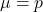and standard deviation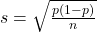Percentage of 70%, sample of 250.

This means that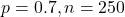Mean: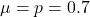Standard deviation: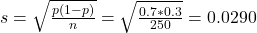The mean of the sampling distribution of p is of 0.7 and the standard deviation 0.029

2.